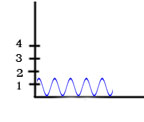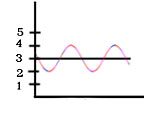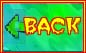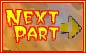Light Basics Types of Light Light Gallery The EM Spectrum

## Amplitude is...The Amplitude is .6The Amplitude is 1
• Amplitude is a measurement of the top (or bottom) half of the wave.

What does it mean?
Waves carry energy, and the amplitude of a wave is generally a measure of how much energy the wave carries. In the same way, the displacement of a bow string, determines the amount of energy that propels the arrow. Energy is a positive quantity, so the amplitude is a positive number.

However...
Scientists don't usually talk about the amplitude of a light wave, since light isn't traveling in some substance like water or air. Instead they talk about the intensity of the light. Intensity is like brightness, and is measured as the rate at which light energy is delivered to a unit of surface, or energy per unit time per unit area. For instance, the intensity of the sunlight on your solar panel, multiplied by the panel's area, would tell you how much power (energy per unit time) you have available to run your solar water heater.

You already know that different types, or wavelengths of light also have different energies. How does intensity fit in? Different wavelengths of light also have more or less energy, depending on whether the wavelength is shorter or longer. So the energy of light depends on both intensity and wavelength.

To sum up,
you now know a lot about waves and light:

• How to measure wavelength
• That light wavelengths are often measured in units of angstroms
• That different wavelengths of light have different energies.
• That intensity is the amount of energy delivered per unit time per unit area.
• That light energy depends on both the brightness and wavelength of the light.Back And you're ready to go on to the light tour. Have Fun!light tour start